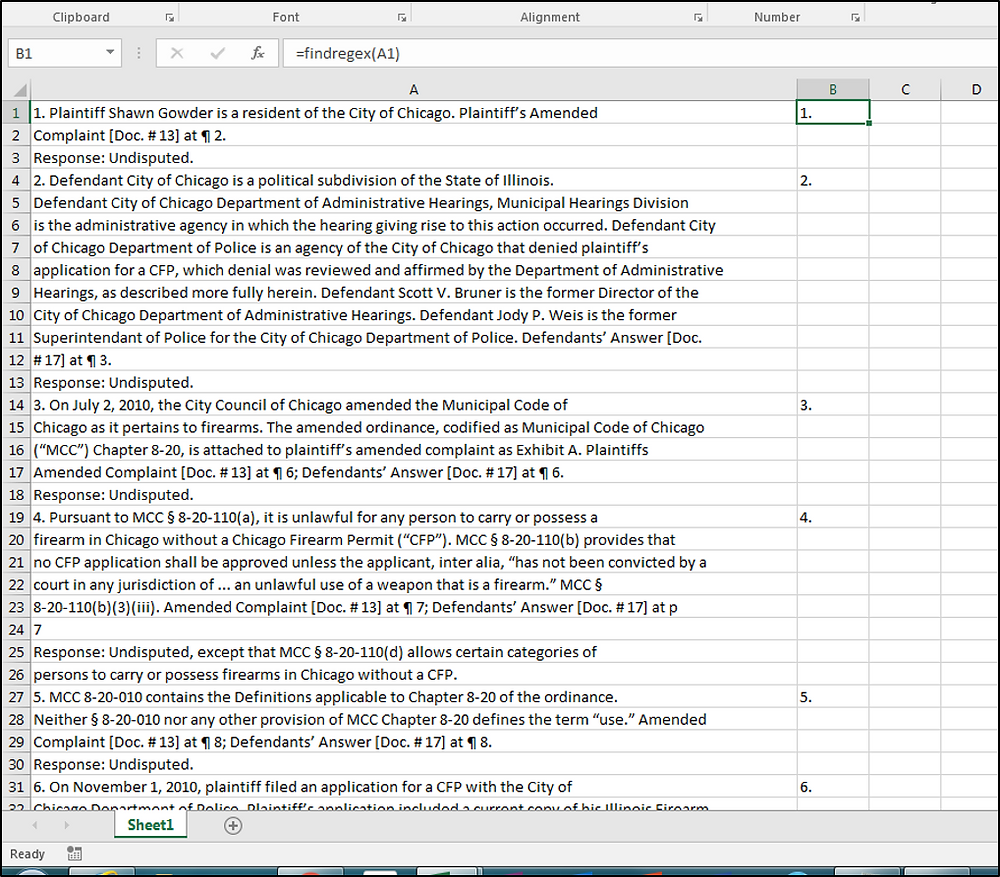Search

# Regular Expression search for text at the beginning of a line - ^

A caret ^ can be used in a RegEx search to specify that you are focusing on strings that start at the beginning of a line of text. In this example I'm showing how this RegEx feature can be employed in the Visual Basic macro to run Regular Expression searches that was the September 2, 2015 Tip of the Night. I've modified the code in this way with the aim of being able to pick out instances of numbered lines (instances where a line begins with a number (whether one digit, two digit, or three digit) followed by a period and a space, or a letter, a period and a space. So this Regex will find

1. Paris

1c. Sofia

22. London

27b. Warsaw

100. Berlin

101a. Rome

. . . and so forth

Before entering this macro be sure to go in Visual Basic to Tools . . . References . . . and check off Microsoft VBScript Regular Expressions 5.5.

Function findRegex(sentance) Dim regEx As New VBScript_RegExp_55.RegExp Dim matches, s regEx.Pattern = "^[0-9]. |^[0-9][0-9]. |^[0-9][0-9][0-9]. |^[0-9][a-z]. |^[0-9][0-9][a-z]. |^[0-9][0-9][0-9][a-z]. " regEx.IgnoreCase = True 'True to ignore case regEx.Global = True 'True matches all occurances, False matches the first occurance s = "" If regEx.Test(sentance) Then Set matches = regEx.Execute(sentance) For Each Match In matches s = s & "" s = s & "" & Match.Value & " " s = s & Chr(10) Next findRegex = s Else findRegex = "" End If End Function

So when we enter the findRegex search that this formula (=findRegex) creates in a column in Excel, we get these results:This is useful in parsing out the statements and responses which are given in a Rule 56.1 Statement of Facts filed for a summary judgment motion. It's easy enough to tag the response with a LEFT formula - =IF(LEFT(A1,10)="Response: ","Response","") - and once you've got the beginning of each statement and each response tagged separating them into separate columns can be accomplished quite easily as I'll explain tomorrow night when I can stay up later . . . ; )